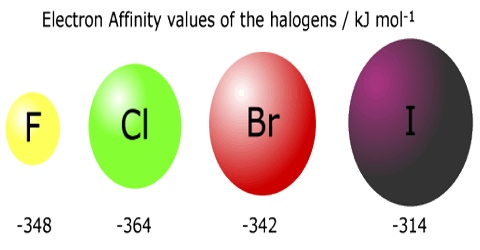Chemistry

# Electron AffinityElectron Affinity

The electron affinity of an atom or molecule is defined as the amount of energy released or spent when an electron is added to a neutral atom or molecule in the gaseous state to form a negative ion. When a neutral atom in the gaseous phase acquires an electron to form a stable anion energy is released. For example;

Cl (g) + e → Cl (g);                           ∆H0 = – ve

This happens because an atom like chlorine can attain a stable noble gas configuration by acquiring an electron. To describe this phenomenon a term electron affinity is defined as below:

The first electron affinity of an element is the enthalpy change when each of one mole of atoms of an element in the gaseous phase acquire one electron to form one mole of uninegatively charged gaseous anions.

As mentioned this process is exothermic. For oxygen the equation is written as;

O (g) + e = O (g);                               ∆H0 = – 141 kJmol-1

When a second electron is to be acquired by s uninegatively charged anion energy has to be added because the second election is to be pushed against the repulsion of the already present extra electron in the atom. The second electron affinity of an element is, therefore, endothermic.

O (g) + e = O2- (g);                             ∆H0 = 710 kJmol-1